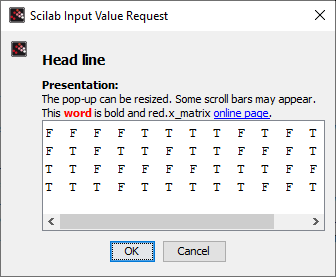Scilab Home page | Wiki | Bug tracker | Forge | Mailing list archives | ATOMS | File exchange
Change language to: English - Français - Português - Русский
Scilabヘルプ >> GUI > x_matrix

x_matrix

Edits a matrix and waits for validation (modal)

Arguments

column vector of text: Text displayed before the matrix. Extended UTF-8 characters are supported. Any element starting with "<body>" can use HTML.4 tags afterward.

matrixIn
Initial vector or matrix of booleans, encoded integers, real or complex numbers, or text.

matrixOut
Modified matrix, of the same type than matrixIn, if "OK" is pressed. Or [] if "Cancel" is pressed.

Description

Edits a matrix in a modal way: Scilab is paused while the matrix is displayed and the user can modify it. One of both buttons "OK" or "Cancel" must be clicked to validate or cancel modifications. Either the modified matrix or [] is returned accordingly. Then Scilab resumes the execution of next instructions.

The pop-up is sized according to the displayed matrix. It can be resized interactively. If it becomes narrower than the matrix or the header, long header lines are wrapped, and some scroll bars appear.

Examples

i = int8(grand(3,4,"uin",-150,150))

// With booleans
b = i > 0
m = x_matrix("<html><body>Matrix of <b>booleans", b)

// With encoded integers
m = x_matrix("<html><body>Matrix of <b>int8 integers", i)

// With real numbers
c = grand(5,4,"uin",-15,15) + grand(5,4,"uin",0,9)/10;
c([14 8 16]) = [%nan %inf -%inf]
m = x_matrix("<html><body>Matrix of <b>decimal numbers", c)

// With complex numbers
c = grand(5,3,"uin",-15,15) + grand(5,3,"uin",-15,15)*%i;
c(2,2) = 3-1.24e-31*%i;
c(4,3) = complex(0,%nan)
m = x_matrix("<body>Matrix of <b>complex numbers", c)

// With texts
t = ["ab" "I don''t" "αβδεϵζ"
"j"  """Yes"""  "àéêèïôûù"]
m = x_matrix("<body>Matrix of <b>text", t)With a multiple-line and styled header:

//
online = "https://help.scilab.org/docs/current/en_US/x_matrix.html";
"<body><b>Presentation:</b>"
"The pop-up can be resized. Some scroll bars may appear."
"<body>This <font color=''red''><b>word</b></font> is bold and red. " + ..
"<body>x_matrix <a href=''" + online + "''>online page</a>."
]
b = grand(4,14,"uin",-2,1) < 0• x_mdialog — 対話的なベクトル/行列入力用のダイアログ.
• x_dialog — 対話的な複数行入力用ダイアログ.
• editvar — Scilab variable editor
• uicontrol table — グラフィックユーザーインターフェイスオブジェクトを作成
• messagebox — メッセージボックスを開く.
• prettyprint — Converts a Scilab object into some corresponding LaTeX, TeX, MathML or HTML strings

History

 バージョン 記述 6.1.1 Booleans, encoded integers, and text are supported. The display of real and complex matrices is improved.